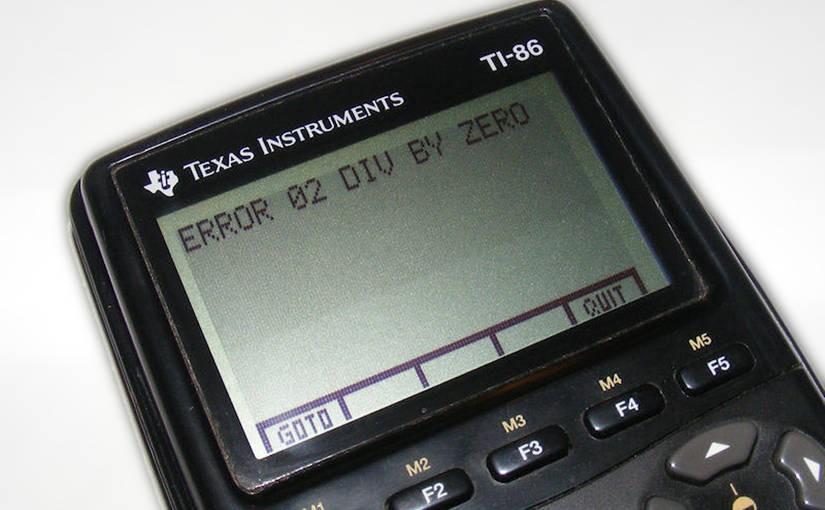# cs193p – Project #2 Assignment #2 Extra Task #3Please note, this blog entry is from a previous course. You might want to check out the current one.

Add a new method, evaluateAndReportErrors(). It should work like evaluate() except that if there is a problem of any kind evaluating the stack (not just unset variables or missing operands, but also divide by zero, square root of a negative number, etc.), instead of returning nil, it will return a String with what the problem is (if there are multiple problems, you can simply return any one of them you wish). Report any such errors in the display of your calculator (instead of just making it blank or showing some weird value). You must still implement evaluate() as specified in the Required Tasks above, but, if you want, you can have evaluate() return nil if there are any errors (not just in the “unset variable” or “not enough operands” case). The push and perform methods should still return Double? (which is kind of a wasted evaluation, but we want to be able to evaluate your Extra Credit separate from the Required Tasks).

The definitions of the unary and binary operations get new parameters to pass optional error-test methods (and all their occurrences need to be adjusted for the new parameter):

```    private enum Op: Printable
{
...
case UnaryOperation(String, Double -&gt; Double, (Double -&gt; String?)?)
case BinaryOperation(String, Int, (Double, Double) -&gt; Double,
((Double, Double) -&gt; String?)?)
...
case .UnaryOperation(let symbol, _, _):
...
case .BinaryOperation(let symbol, _, _, _):
...
case .BinaryOperation(_, let precedence, _, _):
...
}
```

Only the division and the square-root operation receive such error-test methods:

```    init() {
...
learnOp(Op.BinaryOperation(&quot;×&quot;, 2, *, nil))
learnOp(Op.BinaryOperation(&quot;÷&quot;, 2, { \$1 / \$0 },
{ divisor, _ in return divisor == 0.0 ? &quot;Division by Zero&quot; : nil }))
learnOp(Op.BinaryOperation(&quot;+&quot;, 1, +, nil))
learnOp(Op.BinaryOperation(&quot;−&quot;, 1, { \$1 - \$0 }, nil))
learnOp(Op.UnaryOperation(&quot;√&quot;, sqrt, { \$0 &lt; 0 ? &quot;SQRT of Neg. Number&quot; : nil }))
learnOp(Op.UnaryOperation(&quot;sin&quot;, sin, nil))
learnOp(Op.UnaryOperation(&quot;cos&quot;, cos, nil))
learnOp(Op.UnaryOperation(&quot;±&quot;, { -\$0 }, nil))
...
}
```

A new private property will hold the current error message, if there is any:

```    private var error: String?
```

For the unary and binary operations check if an error test is available. If there is an error, set the error property and stop further evaluation. Do the same if a variable has no value set. When the method reaches the end without proper evaluation, there where not enough operands, which is also an error:

```    private func evaluate(ops: [Op]) -&gt; (result: Double?, remainingOps: [Op]) {
...
case .UnaryOperation(_, let operation, let errorTest):
...
if let errorMessage = errorTest?(operand) {
error = errorMessage
return (nil, operandEvaluation.remainingOps)
}
...
case .BinaryOperation(_, _, let operation, let errorTest):
...
if let errorMessage = errorTest?(operand1, operand2) {
error = errorMessage
return (nil, op2Evaluation.remainingOps)
}
...
case .Variable(let symbol):
if let variable = variableValues[symbol] {
return (variableValues[symbol], remainingOps)
}
error = &quot;Variable Not Set&quot;
return (nil, remainingOps)
}
if error == nil {
error = &quot;Not Enough Operands&quot;
}
}
return (nil, ops)
}
```

At the beginning of each evaluation reset the error status:

```    func evaluate() -&gt; Double? {
error = nil
...
}
```

The required new method, just calls the existing evaluation method, returns the result if there is any, and otherwise the error message:

```    func evaluateAndReportErrors() -&gt; AnyObject? {
let (result, _) = evaluate(opStack)
return result != nil ? result : error
}
```

And there are some adjustments left to cope with the additional parameter:

```    private func description(ops: [Op]) -&gt; (result: String?, remainingOps: [Op], precedence: Int?) {
...
case .UnaryOperation(let symbol, _, _):
...
case .BinaryOperation(let symbol, _, _, _):
...
}
```

To display the error message in the calculator view, call the new method when there is no valid result:

```    var displayValue: Double? {
...
set {
if (newValue != nil) {
...
} else {
if let result = brain.evaluateAndReportErrors() as? String {
display.text = result
} else {
display.text = &quot; &quot;
}
}
...
}
}
```

The complete code for extra task #3 is available on GitHub.